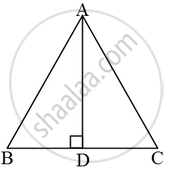# If ABC is an equilateral triangle of side a, prove that its altitude = √3/2a - Mathematics

Sum

If ABC is an equilateral triangle of side a, prove that its altitude =  \frac { \sqrt { 3 } }{ 2 } a

#### Solution

∆ABD is an equilateral triangle.

We are given that AB = BC = CA = a.

Now, in right angled triangles ABD and ACD, we have

AB = AC (Given)

∆ABD ≅ ∆ACD (By RHS congruence)

⇒ BD = CD ⇒ BD = DC = \frac { 1 }{ 2 }BC = \frac { a }{ 2 }From right triangle ABD.

AB^2 = AD^2 + BD^2

=>a^2=AD^2+(a/2)^2

AD^2=a^2-a^2/4=3/4a^2

\Rightarrow AD=\frac{\sqrt{3}}{2}a

Concept: Right-angled Triangles and Pythagoras Property
Is there an error in this question or solution?